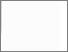# Project risk analysis

Payne, B., 1998. Project risk analysis. PhD, Nottingham Trent University.Preview
Text
10183027.pdf - Published version

## Abstract

This thesis reviews the history and literature of Project Risk Analysis (PRA) and provides a conceptual model where time, cost and quality characteristics are modelled. Investigation of project managers requirements, specifically in the construction industry, reveals the need for a model incorporating the operation performance of the project outcome. The concept of project quality loss is introduced and models explored throughout. A representation of a project as a set of sequential phases is developed which provides the framework for modelling the project operation measures reliability, availability and output.

Investigation of current methods for performing a project risk analysis of a single characteristic such as time, revealed the choice of approach needs to depend on the activity distribution used, the network configuration and the desired accuracy. A Laplace transform approach is developed where the explicit probability function is obtained for project completion time assuming activity distributions are special Erlangian. An algorithm, based on the network reduction method of Dodin (1984a), is provided and implemented with the developed Laplace transform approach within the Mathematica system. Network examples are investigated, including the 'Forbidden Network' configuration of Ringer (1969) and comparisons made to the approaches PERT, method of moments and simulation.

Dependency relationships are investigated between the characteristics time, cost and quality. A bivariate exponential extension distribution is developed to model time-cost dependency of a project phase and a method defined for obtaining the convolution of n such densities. Time-quality loss relationships are discussed and a model developed.

A generalised model of the performance of a project is formulated as a stochastic process where the state space is partly discrete and partly continuous. Examples where the time, cost and quality characteristics are measured and discussion of possible Markov dependencies are presented in addition to a quality loss dependency model using the uniform distribution.

The dependency modelling capabilities of the generalised model are extended and a simulation program is developed to analyse the dependency between the characteristics time, cost and quality loss and the dependency between phases.

Item Type: Thesis
Creators: Payne, B.
Date: 1998
ISBN: 9781369313192
Identifiers:
NumberType
PQ10183027Other
Divisions: Professional Services > Libraries and Learning Resources
Record created by: Linda Sullivan
Date Added: 28 Aug 2020 12:40Edit View